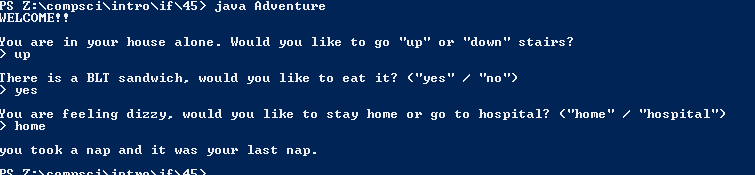## Code

```    /// Name: Caelan Dorstad
/// Period: 6
/// Date Finished: 10/20/2015

import java.util.Scanner;

public static void main(String[] args){

Scanner keyboard = new Scanner(System.in);

String Q1, Q2, Q3, A1 = "up", A2 = "down" , B1 = "yes" , B2 = "no", C1 = "hospital" , C2 = "home", C3 = "finish" ,
C4 = "procrastinate", C5 = "spend", C6 = "save", C7 = "chill" , C8 = "do";

int R1 = 0, R2 = 0 , R3 = 0;

System.out.println ("WELCOME!!");
System.out.println();

System.out.println("You are in your house alone. Would you like to go \"up\" or \"down\" stairs?");
System.out.print("> ");
Q1 = keyboard.next();
System.out.println();

if (Q1.equals(A1)){
R1 += 1;
}

else if (Q1.equals(A2)){
R1 += 2;
}

if (R1 == 1){
System.out.println("There is a BLT sandwich, would you like to eat it? (\"yes\" / \"no\") ");
System.out.print("> ");
Q2 = keyboard.next();
System.out.println();

if (Q2.equals(B1)){
R2 += 1;
}

if (Q2.equals(B2)){
R2 += 2;
}

}

else if (R1 == 2){
System.out.println("There is a laundering machine, would you like to do chores? (\"yes\" / \"no\") ");
System.out.print("> ");
Q2 = keyboard.next();
System.out.println();

if (Q2.equals(B1)){
R2 += 3;
}

else if (Q2.equals(B2)){
R2 += 4;
}

}

if ( R2 == 1  ) {
System.out.println("You are feeling dizzy, would you like to stay home or go to hospital? (\"home\" / \"hospital\") ");
System.out.print("> ");
Q3 = keyboard.next();
System.out.println();

if (Q3.equals(C1)){
System.out.println("Docters found that you were allergic to bacon.");
}

else if (Q3.equals(C2)){
System.out.println("you took a nap and it was your last nap.");
}
}

else if (R2 == 2 ) {
System.out.println("You suddenly found that you have work to do.");
System.out.println("would you finish it or procrastinate until late night? (\"finish\" / \"procrastinate\") ");
System.out.print("> ");
Q3 = keyboard.next();
System.out.println();

if (Q3.equals(C3)){
System.out.println("You finished your work and it contributed to society greatly. You became a billionaire.");
}

else if (Q3.equals(C4)){
System.out.println("You later found that your work was a chance to success, but it was too late. ");
}
}

else if (R2 == 3 ) {
System.out.println("Would you spend that money or save it? (\"spend\" / \"save\")");
System.out.print("> ");
Q3 = keyboard.next();
System.out.println();

if (Q3.equals(C6)){
System.out.println("After 4 months, you found that your mom took your money away and used it to buy Justin Bieber's album.");
}

else if (Q3.equals(C5)){
System.out.println("You were able to buy a guitar and become a superstar singer.");
}
}

else if (R2 == 4 ) {
System.out.println("You are asked to do chores, what would you do? (\"do\" / \"chill\") ");
System.out.print("> ");
Q3 = keyboard.next();
System.out.println();

if (Q3.equals(C7)){
System.out.println("You are grounded.");
}

else if (Q3.equals(C8)){